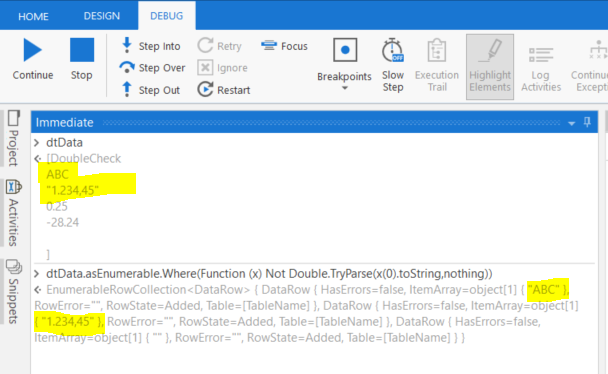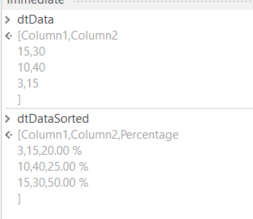# How to sort datatable based on column which has percentage and it is generic value datatype

I have used sort datatable activity to sort for percentage and it is generic value datatype.But I am getting error as compare cannot be implemented for System.decimal with Uipath.core.genericvalue.

Thanks,
Nawaz.

Hi

Before sorting out we need to convert that type to decimal in the datatable

With this expression

dt = (From row In dt.Select Order By Convert.ToDecimal(if(String.IsNullOrEmpty(row(“yourcolumnname”).ToString),“0”,row(“yourcolumnname”).ToString)) Ascending Select row).ToArray.CopyToDatatable()

Cheers @Mohammed_Nawazuddin

Hi Palaniyappan,

I have used the same expression as mentioned, But it is saying input string was not in a correct format.

Can you pls let me know what went wrong

Thanks,
Nawaz.

Can I have the screenshot of the error if possible

@Mohammed_Nawazuddin

Hi,

Plz find the error screenshot

Thanks,
Nawaz.

Is there any special characters along the value like minus -
@Mohammed_Nawazuddin

Hi,

No Value as -

we can do some analysis on the data for this:

• set a breakpoint on the activity which is failing
• debug and get paused
• open immediate panel
• run following statement:
YourDataTableVar.asEnumerable.Where(Function (x) Not Double.TryParse(x(YourColNameOrIndex).toString,nothing))

share the result with us, so we can inspect

It will report the rows, where the column is failing for getting parsed into Double/Decimal

like this:Can you also confirm that you are working on Studio / Studio Pro, but not on StudioX? Thanks

Hi,

The above function is not working in immediate panel and I am working on studio Pro.
I need to convert Generic Value type to decimal type use sort datatable activity.

Thanks,
Nawaz.

what was done, what was the issue?

I have two columns in excel and I wanted to get the percentage from those two columns.So I have created generic value variable and divided those two columns and got the decimal value.Then I used format value activity to convert to Percentage and added that percentage as a new column in datatable and now I want to sort based on the Percentage column as descending order and I am using Sort Datatable activity but I am getting error as cannot be implemented for System.decimal with Uipath.core.genericvalue.
Finally I want to save the whole data in excel

I hope you understood the issue.

Thanks,
Nawaz.

The question was more related to

what was done within the immediate panel.

But your explanation did help very much.
Maybe Following scenario will be similar to your case:

Variables:Flow:LINQ:

``````(From d In dtData.AsEnumerable
Let p1 =CDbl(d(0).toString) / CDbl(d(1).toString)
Let ps = p1.ToString("P2")
Let ra = New Object(){d(0), d(1), ps}
Order By p1
``````

input / output:feel free to adopt var names and ColumnNames or Indexes as by your settings

Hi Peter,

This code ihas given me all scenarios .Thanks for your effort. Just one help from the Linq code as I need to get the percentage in descending order. In Linq code what needs to be changed as I am new into Uipath with less coding background. It would be helpful.

Thanks,
Nawaz.

@Mohammed_Nawazuddin we use DESCENDING for this

``````(From d In dtData.AsEnumerable
Let p1 =CDbl(d(0).toString) / CDbl(d(1).toString)
Let ps = p1.ToString("P2")
Let ra = New Object(){d(0), d(1), ps}
Order By p1 Descending
``````

Hi Peter,

Thanks for your quick reply and it found very helpful. Just need to add one more condition in the Linq code .I need to update the data which are only above 20% in the excel sheet.It would be helpful if you can add a if condition to the exisiting linq code to update only above 20% in the code.

Thanks,
Nawaz.

not sure what is meant in detail. With the assumption that only rows are to handle with % > 20 give a try on following:

``````(From d In dtData.AsEnumerable
Let p1 =CDbl(d(0).toString) / CDbl(d(1).toString)
Where p1 > 20
Let ps = p1.ToString("P2")
Let ra = New Object(){d(0), d(1), ps}
Order By p1 Descending
``````

Hi Peter,
Thanks for quick response. I have tried the above code for %>20 but it has thrown error saying the source has no data rows. Any suggestions would be helpful or or any code changes.

Thanks,
Nawaz.

this happens when filter result is empty we can handle defensive by following:

Assign activity
left side: drResult | DataType: List(Of DataRow)
Right side:

``````(From d In dtData.AsEnumerable
Let p1 =CDbl(d(0).toString) / CDbl(d(1).toString)
Where p1 > 20
Let ps = p1.ToString("P2")
Let ra = New Object(){d(0), d(1), ps}
Order By p1 Descending
``````

Depending on drResult.Count > 0
Then: dtFiltered = drResult.CopyToDataTable
Else: dtFiltered = dtData.Clone

Hi Peter,

As suggested I have used list(Datarow) but its throwing an error as ‘rows’ is not a member of sysytem.collections.generic.list(syytem.data.datarow

Kindly note we applied this pattern very often sucessfully in production projects.

plese ensure that
dtDataSorted is of datatype: datatable
drResult is of DataType: List(Of DataRow)